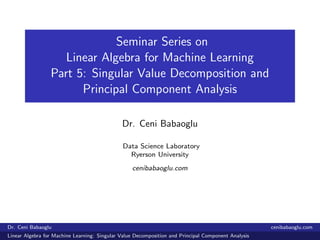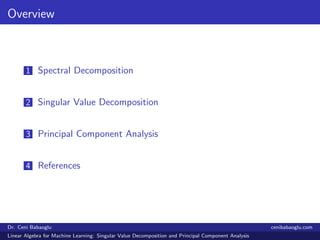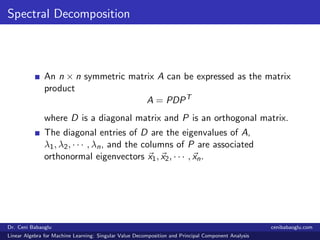Successfully reported this slideshow.

# 5. Linear Algebra for Machine Learning: Singular Value Decomposition and Principal Component Analysis×

# 5. Linear Algebra for Machine Learning: Singular Value Decomposition and Principal Component Analysis

The seminar series will focus on the mathematical background needed for machine learning. The first set of the seminars will be on "Linear Algebra for Machine Learning". Here are the slides of the fifth part which is discussing singular value decomposition and principal component analysis.
Here are the slides of the first part which was discussing linear systems: https://www.slideshare.net/CeniBabaogluPhDinMat/linear-algebra-for-machine-learning-linear-systems/1
Here are the slides of the second part which was discussing basis and dimension:
https://www.slideshare.net/CeniBabaogluPhDinMat/2-linear-algebra-for-machine-learning-basis-and-dimension
Here are the slides of the third part which is discussing factorization and linear transformations.
https://www.slideshare.net/CeniBabaogluPhDinMat/3-linear-algebra-for-machine-learning-factorization-and-linear-transformations-130813437
Here are the slides of the fourth part which is discussing eigenvalues and eigenvectors.
https://www.slideshare.net/CeniBabaogluPhDinMat/4-linear-algebra-for-machine-learning-eigenvalues-eigenvectors-and-diagonalization

The seminar series will focus on the mathematical background needed for machine learning. The first set of the seminars will be on "Linear Algebra for Machine Learning". Here are the slides of the fifth part which is discussing singular value decomposition and principal component analysis.
Here are the slides of the first part which was discussing linear systems: https://www.slideshare.net/CeniBabaogluPhDinMat/linear-algebra-for-machine-learning-linear-systems/1
Here are the slides of the second part which was discussing basis and dimension:
https://www.slideshare.net/CeniBabaogluPhDinMat/2-linear-algebra-for-machine-learning-basis-and-dimension
Here are the slides of the third part which is discussing factorization and linear transformations.
https://www.slideshare.net/CeniBabaogluPhDinMat/3-linear-algebra-for-machine-learning-factorization-and-linear-transformations-130813437
Here are the slides of the fourth part which is discussing eigenvalues and eigenvectors.
https://www.slideshare.net/CeniBabaogluPhDinMat/4-linear-algebra-for-machine-learning-eigenvalues-eigenvectors-and-diagonalization

### 5. Linear Algebra for Machine Learning: Singular Value Decomposition and Principal Component Analysis

1. 1. Seminar Series on Linear Algebra for Machine Learning Part 5: Singular Value Decomposition and Principal Component Analysis Dr. Ceni Babaoglu Data Science Laboratory Ryerson University cenibabaoglu.com Dr. Ceni Babaoglu cenibabaoglu.com Linear Algebra for Machine Learning: Singular Value Decomposition and Principal Component Analysis
2. 2. Overview 1 Spectral Decomposition 2 Singular Value Decomposition 3 Principal Component Analysis 4 References Dr. Ceni Babaoglu cenibabaoglu.com Linear Algebra for Machine Learning: Singular Value Decomposition and Principal Component Analysis
3. 3. Spectral Decomposition An n × n symmetric matrix A can be expressed as the matrix product A = PDPT where D is a diagonal matrix and P is an orthogonal matrix. The diagonal entries of D are the eigenvalues of A, λ1, λ2, · · · , λn, and the columns of P are associated orthonormal eigenvectors x1, x2, · · · , xn. Dr. Ceni Babaoglu cenibabaoglu.com Linear Algebra for Machine Learning: Singular Value Decomposition and Principal Component Analysis
4. 4. Spectral Decomposition The expression A = PDPT is called the spectral decomposition of A. We can write it as A = x1 x2 · · · xn         λ1 0 · · · · · · 0 0 λ2 0 · · · 0 ... 0 ... ... 0 ... ... ... ... 0 0 0 · · · 0 λn              xT 1 xT 2 ... xT n      Dr. Ceni Babaoglu cenibabaoglu.com Linear Algebra for Machine Learning: Singular Value Decomposition and Principal Component Analysis
5. 5. Spectral Decomposition The matrix product DPT gives DPT =         λ1 0 · · · · · · 0 0 λ2 0 · · · 0 ... 0 ... ... 0 ... ... ... ... 0 0 0 · · · 0 λn              xT 1 xT 2 ... xT n      =      λ1xT 1 λ2xT 2 ... λnxT n      A = x1 x2 · · · xn      λ1xT 1 λ2xT 2 ... λnxT n      Dr. Ceni Babaoglu cenibabaoglu.com Linear Algebra for Machine Learning: Singular Value Decomposition and Principal Component Analysis
6. 6. Spectral Decomposition We can express A as a linear combination of the matrices xj xT j , and the coeﬃcients are the eigenvalues of A, A = n j=1 λj xj xT j = λ1x1xT 1 + λ2x2xT 2 + · · · + λnxnxT n . Dr. Ceni Babaoglu cenibabaoglu.com Linear Algebra for Machine Learning: Singular Value Decomposition and Principal Component Analysis
7. 7. Singular Value Decomposition Singular Value Decomposition is based on a theorem from linear algebra which says the following: a rectangular matrix A can be broken down into the product of three matrices: an orthogonal matrix U, a diagonal matrix S, the transpose of an orthogonal matrix V . Dr. Ceni Babaoglu cenibabaoglu.com Linear Algebra for Machine Learning: Singular Value Decomposition and Principal Component Analysis
8. 8. Singular Value Decomposition Let A be an m × n real matrix. Then there exist orthogonal matrices U of size m × m and V of size n × n such that A = USV T where S is an m × n matrix with nondiagonal entries all zero and s11 ≥ s12 ≥ · · · ≥ spp ≥ 0, p = min{m, n}. the diagonal entries of S are called the singular values of A, the columns of U are called the left singular vectors of A, the columns of V are called the right singular vectors of A, the factorization USV T is called the singular value decomposition of A. Dr. Ceni Babaoglu cenibabaoglu.com Linear Algebra for Machine Learning: Singular Value Decomposition and Principal Component Analysis
9. 9. Singular Value Decomposition A = USV T , UT U = I, V T V = I, the columns of U are orthonormal eigenvectors of AAT , the columns of V are orthonormal eigenvectors of AT A, S is a diagonal matrix containing the square roots of eigenvalues from U or V in descending order. Dr. Ceni Babaoglu cenibabaoglu.com Linear Algebra for Machine Learning: Singular Value Decomposition and Principal Component Analysis
10. 10. Example Let’s ﬁnd the singular value decomposition of A= 3 1 1 −1 3 1 . In order to ﬁnd U, we start with AAT . AAT = 3 1 1 −1 3 1   3 −1 1 3 1 1   = 11 1 1 11 Eigenvalues: λ1 = 12 and λ2 = 10. Eigenvectors: u1 = 1 1 and u2 = 1 −1 . Dr. Ceni Babaoglu cenibabaoglu.com Linear Algebra for Machine Learning: Singular Value Decomposition and Principal Component Analysis
11. 11. Example Using Gram-Schmidt Process v1 = u1 w1 = v1 |v1| = 1/ √ 2 1/ √ 2 v2 = u2 − (u2, v1) (v1, v1) v1 v2 = 1 −1 − 0 1 1 = 1 −1 − [0, 0] = 1 −1 w2 = v2 |v2| = 1 √ 2 , −1 √ 2 U = 1/ √ 2 1/ √ 2 1/ √ 2 −1/ √ 2 Dr. Ceni Babaoglu cenibabaoglu.com Linear Algebra for Machine Learning: Singular Value Decomposition and Principal Component Analysis
12. 12. Example The calculation of V is similar. V is based on AT A, so we have AT A = 3 −1 1 3 1 1 3 1 1 −1 3 1 = 10 0 2 0 10 4 2 4 2 We ﬁnd the eigenvalues of AT A Eigenvalues: λ1 = 12, λ2 = 10 and λ3 = 0. Eigenvectors: u1 = 1 2 1 , u2 = 2 −1 0 and u3 = 1 2 −5 . Dr. Ceni Babaoglu cenibabaoglu.com Linear Algebra for Machine Learning: Singular Value Decomposition and Principal Component Analysis
13. 13. Example v1 = u1, w1 = v1 |v1| = 1 √ 6 , 2 √ 6 , 1 √ 6 v2 = u2 − (u2, v1) (v1, v1) v1 = [2, −1, 0] w2 = v2 |v2| = 2 √ 5 , −1 √ 5 , 0 Dr. Ceni Babaoglu cenibabaoglu.com Linear Algebra for Machine Learning: Singular Value Decomposition and Principal Component Analysis
14. 14. Example v3 = u3 − (u3, v1) (v1, v1) v1 − (u3, v2) (v2, v2) v2 = −2 3 , −4 3 , 10 3 w3 = v3 |v3| = 1 √ 30 , 2 √ 30 , −5 √ 30 V =    1√ 6 2√ 5 1√ 30 2√ 6 −1√ 5 2√ 30 1√ 6 0 −5√ 30    , V T =    1√ 6 2√ 6 1√ 6 2√ 5 −1√ 5 0 1√ 30 2√ 30 −5√ 30    Dr. Ceni Babaoglu cenibabaoglu.com Linear Algebra for Machine Learning: Singular Value Decomposition and Principal Component Analysis
15. 15. Example A = USV T A = 1√ 2 1√ 2 1√ 2 −1√ 2 √ 12 0 0 0 √ 10 0   1√ 6 2√ 6 1√ 6 2√ 5 −1√ 5 0 1√ 30 2√ 30 −5√ 30   = 3 1 1 −1 3 1 Dr. Ceni Babaoglu cenibabaoglu.com Linear Algebra for Machine Learning: Singular Value Decomposition and Principal Component Analysis
16. 16. Principal Component Analysis Let λ1, λ2, · · · , λn be the eigenvalues of A and x1, x2, · · · , xn be a set of associated orthonormal eigenvectors. Then the spectral decomposition of A is given by A = λ1x1xT 1 + λ2x2xT 2 + · · · + λnxnxT n . If A is a real n × n matrix with real eigenvalues λ1, λ2, · · · , λn, then an eigenvalue of largest magnitude is called a dominant eigenvalue of A. Dr. Ceni Babaoglu cenibabaoglu.com Linear Algebra for Machine Learning: Singular Value Decomposition and Principal Component Analysis
17. 17. Principal Component Analysis Let X be the multivariate data matrix X =         x11 x12 · · · x1k · · · x1p x21 x22 · · · x2k · · · x2p ... ... ... ... xj1 xj2 · · · xjk · · · xjp ... ... ... ... xn1 xn2 · · · xnk · · · xnp         . The measure of association between the ith and kth variables in the multivariate data matrix is given by the sample covariance sik = 1 n n j=1 (xji − xi ) (xjk − xk) , i = 1, 2, . . . , p, k = 1, 2, . . . , p. Dr. Ceni Babaoglu cenibabaoglu.com Linear Algebra for Machine Learning: Singular Value Decomposition and Principal Component Analysis
18. 18. Principal Component Analysis Let Sn be the p × p covariance matrix associated with the multivariate data matrix X. Sn =      s11 s12 · · · s1p s21 s22 · · · s2p ... ... ... ... sp1 sp2 · · · spp      Let the eigenvalues of Sn be λj , j = 1, 2, . . . , p, where λ1 ≥ λ2 ≥ · · · ≥ λp ≥ 0 and let the associated orthonormal eigenvectors be uj , j = 1, 2, . . . , p. The ith principal component yi is given by the linear combination of the columns of X, where the coeﬃcients are the entries of the eigenvector ui , yi = Xui Dr. Ceni Babaoglu cenibabaoglu.com Linear Algebra for Machine Learning: Singular Value Decomposition and Principal Component Analysis
19. 19. Principal Component Analysis The variance of yi is λi , The covariance of yi and yk, i = k, is zero. If some of the eigenvalues are repeated, then the choices of the associated eigenvectors are not unique; hence the principal components are not unique.     Proportion of the total variance due to the k th principal component     = λk λ1 + λ2 + · · · + λp , k = 1, 2, . . . , p Dr. Ceni Babaoglu cenibabaoglu.com Linear Algebra for Machine Learning: Singular Value Decomposition and Principal Component Analysis
20. 20. Example Let’s compute the ﬁrst and second principal components for the multivariate data matrix X given by X =         39 21 59 28 18 10 21 13 14 13 22 10         . We ﬁrst ﬁnd the sample means x1 ≈ 28.8 and x2 ≈ 15.8. Then we take the matrix of sample means as x = 28.8 15.8 Dr. Ceni Babaoglu cenibabaoglu.com Linear Algebra for Machine Learning: Singular Value Decomposition and Principal Component Analysis
21. 21. Example The variances are s11 ≈ 243.1 and s22 ≈ 43.1 while the covariances are s12 = s21 ≈ 97.8. We take the covariance matrix as Sn = 243.1 97.8 97.8 43.1 Dr. Ceni Babaoglu cenibabaoglu.com Linear Algebra for Machine Learning: Singular Value Decomposition and Principal Component Analysis
22. 22. Example Eigenvalues: λ1 = 282.9744 and λ2 = 3.2256, Eigenvectors: u1 = 0.9260 0.3775 and u2 = 0.3775 −0.9260 . We ﬁnd the ﬁrst principal component as y1 = 0.9260 col1(X) + 0.3775 col2(X). It follows that y1 accounts for the proportion λ1 λ1 + λ2 ( about 98.9%) of the total variance of X. Dr. Ceni Babaoglu cenibabaoglu.com Linear Algebra for Machine Learning: Singular Value Decomposition and Principal Component Analysis
23. 23. Example We ﬁnd the second principal component as y2 = 0.3775 col1(X) − 0.9260 col2(X). It follows that y2 accounts for the proportion λ2 λ1 + λ2 ( about 0.011%) of the total variance of X. Dr. Ceni Babaoglu cenibabaoglu.com Linear Algebra for Machine Learning: Singular Value Decomposition and Principal Component Analysis
24. 24. References Linear Algebra With Applications, 7th Edition by Steven J. Leon. Elementary Linear Algebra with Applications, 9th Edition by Bernard Kolman and David Hill. Dr. Ceni Babaoglu cenibabaoglu.com Linear Algebra for Machine Learning: Singular Value Decomposition and Principal Component Analysis